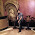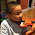## Thursday, 22 October 2015

### Create own HashMap

1. Data Structure need to store the Store the key-value pair.
Make an Entry class to store the HashMap Entries.
Variable: key, value and next
next (Entry) variable is used to avoid the collision of hashmap using chaining (linked list).

2. put() method to put new entries in hashmap.
Identify the bucket using hash (hashcode%SIZE)
a. If no element exists in that bucket: put it as new Entry.

b. If element already exists:
If element is duplicate, replace old one, otherwise find the last element in the chain and add new entry to next pointer of last element.

3. get() method :  return the element in the hashmap
Identify the element bucket by calculate the hash (hashcode%SIZE) of the key, and return the element using equals method.

4. Define size in power on 2
Rehashing is good when the size is exponential of 2.
Set initial size = 2^4 = 16.
To expanding HashMap, make size to its double using power, i.e. 2^5= 32.
To reducing HashMap, make size to its half using power, i.e. 2^3= 8.

public class MyHashMap {
// for better re-sizing is taken as 2^4
private static final int SIZE = 16;

private Entry table[] = new Entry[SIZE];

/**
* To store the Map data in key and value pair.
* Used linked list approach to avoid the collisions
*/
class Entry {
final String key;
String value;
Entry next;

Entry(String k, String v) {
key = k;
value = v;
}

public String getValue() {
return value;
}

public void setValue(String value) {
this.value = value;
}

public String getKey() {
return key;
}
}

/**
* Returns the entry mapped to key in the HashMap.
*/
public Entry get(String k) {
int hash = k.hashCode() % SIZE;
Entry e = table[hash];

// Bucket is identified by hashCode and traversed the bucket
while(e != null) {
if(e.key.equals(k)) {
return e;
}
e = e.next;
}
return null;
}

/**
* If the map previously contained a mapping for the key, the old
* value is replaced.
*/
public void put(String k, String v) {
int hash = k.hashCode() % SIZE;
Entry e = table[hash];

if(e != null) {
// If we will insert duplicate key-value pair,
// Old value will be replaced by new one.
if(e.key.equals(k)) {
e.value = v;
} else {
// Collision: insert new element at the end of list
// in the same bucket
while(e.next != null) {
e = e.next;
}
Entry entryInOldBucket = new Entry(kv);
e.next = entryInOldBucket;
}
} else {
// create new bucket for new element in the map.
Entry entryInNewBucket = new Entry(kv);
table[hash] = entryInNewBucket;
}
}

public static void main(String[] args) {
MyHashMap myHashMap = new MyHashMap();

myHashMap.put("Rahul""SSE");
myHashMap.put("Sattu""SE");
myHashMap.put("Gaurav""SE");

Entry e = myHashMap.get("Awadh");
System.out.println(""+e.getValue());
}
}
Output:SSE

1.This code will not compile itself.

1.It is running Mam.

If you really facing any error please rectify it so we will resolve it.

Running code is:

public class MyHashMap {
// for better re-sizing is taken as 2^4
private static final int SIZE = 16;

private Entry table[] = new Entry[SIZE];

/**
* To store the Map data in key and value pair.
* Used linked list approach to avoid the collisions
*/
class Entry {
final String key;
String value;
Entry next;

Entry(String k, String v) {
key = k;
value = v;
}

public String getValue() {
return value;
}

public void setValue(String value) {
this.value = value;
}

public String getKey() {
return key;
}
}

/**
* Returns the entry mapped to key in the HashMap.
*/
public Entry get(String k) {
int hash = k.hashCode() % SIZE;
Entry e = table[hash];

// Bucket is identified by hashCode and traversed the bucket
while(e != null) {
if(e.key.equals(k)) {
return e;
}
e = e.next;
}
return null;
}

/**
* If the map previously contained a mapping for the key, the old
* value is replaced.
*/
public void put(String k, String v) {
int hash = k.hashCode() % SIZE;
Entry e = table[hash];

if(e != null) {
// If we will insert duplicate key-value pair,
// Old value will be replaced by new one.
if(e.key.equals(k)) {
e.value = v;
} else {
// Collision: insert new element at the end of list
// in the same bucket
while(e.next != null) {
e = e.next;
}
Entry entryInOldBucket = new Entry(k, v);
e.next = entryInOldBucket;
}
} else {
// create new bucket for new element in the map.
Entry entryInNewBucket = new Entry(k, v);
table[hash] = entryInNewBucket;
}
}

public static void main(String[] args) {
MyHashMap myHashMap = new MyHashMap();

myHashMap.put("Rahul", "SSE");
myHashMap.put("Sattu", "SE");
myHashMap.put("Gaurav", "SE");

Entry e = myHashMap.get("Awadh");
System.out.println(""+e.getValue());
}
}

2.It is working as expected

3.I tired to modify the map to be generic, but I can not fix a warning of "private Entry table[] = new Entry[SIZE];" without @SuppressWarnings("unchecked")

package test;

public class MyHashMap {
private static final int SIZE = 16;

private Entry table[] = new Entry[SIZE];

/**
* To store the Map data in key and value pair.
* Used linked list approach to avoid the collisions
*/
static class Entry {
final K key;
V value;
Entry next;

Entry(K k, V v) {
key = k;
value = v;
}

public V getValue() {
return value;
}

public void setValue(V value) {
this.value = value;
}

public K getKey() {
return key;
}
}

/**
* Returns the entry mapped to key in the HashMap.
*/
public Entry get(String k) {
int hash = k.hashCode() % SIZE;
Entry e = table[hash];

// Bucket is identified by hashCode and traversed the bucket
while(e != null) {
if(e.key.equals(k)) {
return e;
}
e = e.next;
}
return null;
}

/**
* If the map previously contained a mapping for the key, the old
* value is replaced.
*/
public void put(K k, V v) {
int hash = k.hashCode() % SIZE;
Entry e = table[hash];

if(e != null) {
// If we will insert duplicate key-value pair,
// Old value will be replaced by new one.
if(e.key.equals(k)) {
e.value = v;
} else {
// Collision: insert new element at the end of list
// in the same bucket
while(e.next != null) {
e = e.next;
}
Entry entryInOldBucket = new Entry(k, v);
e.next = entryInOldBucket;
}
} else {
// create new bucket for new element in the map.
Entry entryInNewBucket = new Entry(k, v);
table[hash] = entryInNewBucket;
}
}

public static void main(String[] args) {
MyHashMap myHashMap = new MyHashMap();